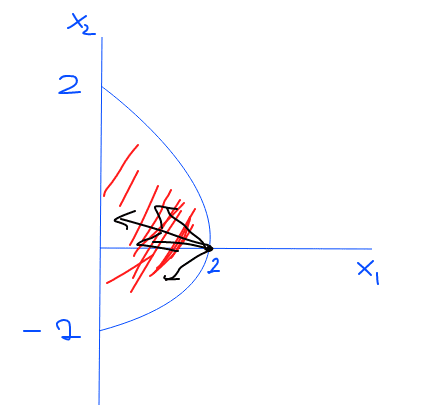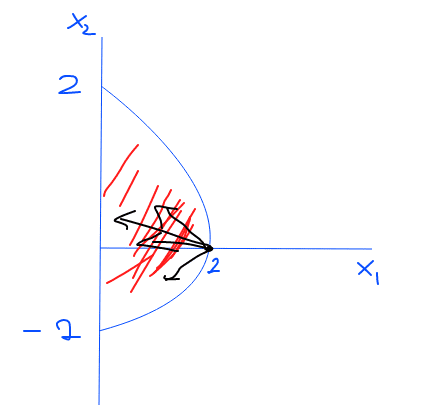# Feasible Directions & FONC

Gold Member

## Homework Statement

$$\text{Minimize } f(x)$$
$$\text{Subject to } \Omega$$

where $$f:R^2 → R \text{ is given by } f(x) = -3x_1 \text{ where } x = [2,0]^\top \text{ and } \Omega = \{x: x_1 + 2x_2^2 \leq 2\}$$

$$\text{Does the point } x^* = [2,0]^\top \text{satisfy F.O.N.C?}$$

## Homework Equations

$$d^T\nabla{f(x^*)} \geq 0$$

has to be satisfied for FONC.

## The Attempt at a Solution

I know FONC, but what I'm having trouble understanding is determining feasible directions. For example, in this case, the point is on the boundary.

$$x + ad = [2,0]^\top + a [d_1, d_2]^\top$$

Now, applying the constraint, I have

$$2ad_1 + 2(ad_2)^2 \leq 0$$

From there, it seems like d_2 is an arbitrary choice, while d_1 has to be chosen accordingly. But, also, since the point is on the corner of the boundary, doesn't that mean that d_2 has to be in some specific direction as well?

Ray Vickson
Homework Helper
Dearly Missed

## Homework Statement

$$\text{Minimize } f(x)$$
$$\text{Subject to } \Omega$$

where $$f:R^2 → R \text{ is given by } f(x) = -3x_1 \text{ where } x = [2,0]^\top \text{ and } \Omega = \{x: x_1 + 2x_2^2 \leq 2\}$$

$$\text{Does the point } x^* = [2,0]^\top \text{satisfy F.O.N.C?}$$

## Homework Equations

$$d^T\nabla{f(x^*)} \geq 0$$

has to be satisfied for FONC.

## The Attempt at a Solution

I know FONC, but what I'm having trouble understanding is determining feasible directions. For example, in this case, the point is on the boundary.

$$x + ad = [2,0]^\top + a [d_1, d_2]^\top$$

Now, applying the constraint, I have

$$2ad_1 + 2(ad_2)^2 \leq 0$$

From there, it seems like d_2 is an arbitrary choice, while d_1 has to be chosen accordingly. But, also, since the point is on the corner of the boundary, doesn't that mean that d_2 has to be in some specific direction as well?

What does the term "FONC" mean? I get the "FO" = "first-order" part, but what is NC?

Gold Member
What does the term "FONC" mean? I get the "FO" = "first-order" part, but what is NC?
Hi Ray. It's First Order Necessary Condition.

Ray Vickson
Homework Helper
Dearly Missed

## Homework Statement

$$\text{Minimize } f(x)$$
$$\text{Subject to } \Omega$$

where $$f:R^2 → R \text{ is given by } f(x) = -3x_1 \text{ where } x = [2,0]^\top \text{ and } \Omega = \{x: x_1 + 2x_2^2 \leq 2\}$$

$$\text{Does the point } x^* = [2,0]^\top \text{satisfy F.O.N.C?}$$

## Homework Equations

$$d^T\nabla{f(x^*)} \geq 0$$

has to be satisfied for FONC.

## The Attempt at a Solution

I know FONC, but what I'm having trouble understanding is determining feasible directions. For example, in this case, the point is on the boundary.

$$x + ad = [2,0]^\top + a [d_1, d_2]^\top$$

Now, applying the constraint, I have

$$2ad_1 + 2(ad_2)^2 \leq 0$$

From there, it seems like d_2 is an arbitrary choice, while d_1 has to be chosen accordingly. But, also, since the point is on the corner of the boundary, doesn't that mean that d_2 has to be in some specific direction as well?

I suggest you draw some feasible directions ##\vec{d} = [d_1,d_2]##. Remember that a point of the form ##\vec{x} = [2,0] + t \vec{d}## only need to satisfy the constraint for small, positive ##t##. In particular, if ##d_1=0## then also you need ##d_2 = 0##. Also, of course, you cannot have ##d_1 > 0##; why not?

Gold Member
I suggest you draw some feasible directions ##\vec{d} = [d_1,d_2]##. Remember that a point of the form ##\vec{x} = [2,0] + t \vec{d}## only need to satisfy the constraint for small, positive ##t##. In particular, if ##d_1=0## then also you need ##d_2 = 0##. Also, of course, you cannot have ##d_1 > 0##; why not?

When I imagine (draw), a coordinate system, with x and y, with our point on the corner of the boundary, I'm inclined to say both ##d_1## and ##d_2## needs to be nonnegative, because otherwise ##d## wouldn't be a feasible direction.

However, as I've said, if I apply ##d## to the constraint inequality, I only get ##d_1 \geq0##

For example, if I had the same constraints, but this time our point was only on the boundary (not necessarily corner), I could see why it'd be only ##d_1 \geq0##. But I'm having trouble understanding this.

Ray Vickson
Homework Helper
Dearly Missed
When I imagine (draw), a coordinate system, with x and y, with our point on the corner of the boundary, I'm inclined to say both ##d_1## and ##d_2## needs to be nonnegative, because otherwise ##d## wouldn't be a feasible direction.

However, as I've said, if I apply ##d## to the constraint inequality, I only get ##d_1 \geq0##

For example, if I had the same constraints, but this time our point was only on the boundary (not necessarily corner), I could see why it'd be only ##d_1 \geq0##. But I'm having trouble understanding this.

By definition, ##\vec{d} = [d_1,d_2]## is a feasible direction at ##\vec{x}_0## if ##\vec{x} = \vec{x}_0 + t \vec{d}## is feasible for all small enough ##t > 0## (note the sign!). So, for ##\vec{x}_0 = [2,0]##, is ##\vec{d} = [1,0]## feasible? Is ##\vec{d} = [1,1]## feasible? Is ##\vec{d} = [1, -0.01]## feasible? Is ##\vec{d} = [-1,10]## feasible? What about ##\vec{d} = [-1, -1/2]?##

Gold Member
By definition, ##\vec{d} = [d_1,d_2]## is a feasible direction at ##\vec{x}_0## if ##\vec{x} = \vec{x}_0 + t \vec{d}## is feasible for all small enough ##t > 0## (note the sign!). So, for ##\vec{x}_0 = [2,0]##, is ##\vec{d} = [1,0]## feasible? Is ##\vec{d} = [1,1]## feasible? Is ##\vec{d} = [1, -0.01]## feasible? Is ##\vec{d} = [-1,10]## feasible? What about ##\vec{d} = [-1, -1/2]?##

Okay, so, if I were to use the definition, I'd say only ## d = [-1, 10] ## and ## d = [-1, -1/2 ] ## are feasible. However, when I'm trying to picture it, I get the following:with the blue curve and line [2,-2] the set red lines inside of the set, I think the feasible directions have to be inside the set because we're right at the corner. So by that logic

## d = [1,0] ## and ## d = [1,1] ##are also feasible. I think either I'm drawing something incorrect, or I just simply can't interpret it.

Ray Vickson
Homework Helper
Dearly Missed
Okay, so, if I were to use the definition, I'd say only ## d = [-1, 10] ## and ## d = [-1, -1/2 ] ## are feasible. However, when I'm trying to picture it, I get the following:with the blue curve and line [2,-2] the set red lines inside of the set, I think the feasible directions have to be inside the set because we're right at the corner. So by that logic

## d = [1,0] ## and ## d = [1,1] ##are also feasible. I think either I'm drawing something incorrect, or I just simply can't interpret it.

If you go any positive distance at all along ##\vec{d} = [1,0]## you go into the infeasible region (the unshaded region in your drawing)! Same for ##\vec{d} = [1,1]##.

•Nugso
Gold Member
If you go any positive distance at all along ##\vec{d} = [1,0]## you go into the infeasible region (the unshaded region in your drawing)! Same for ##\vec{d} = [1,1]##.

Ohh! I think I got it now! Thanks!